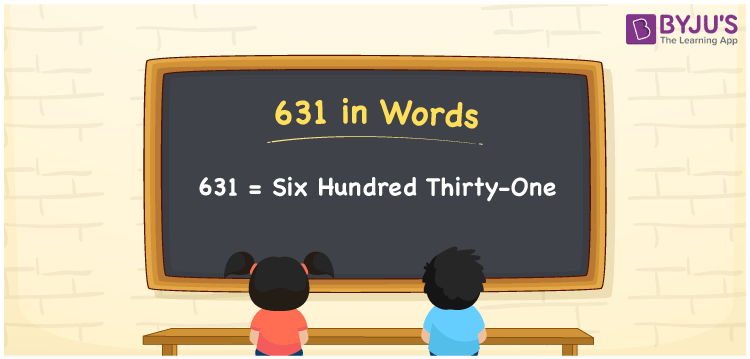# 631 in words

631 in words is written as Six Hundred and Thirty One. 631 represents the count or value. The article on Counting Numbers can give you an idea about count or counting. The number 631 is used in expressions that relate to money, distance, length, year and others. Let us consider an example for 631. ”Six Hundred and Thirty One refugees were welcomed at a temple for food and shelter”.

 631 in words Six Hundred and Thirty One Six Hundred and Thirty One in Numbers 631

## 631 in English Words## How to Write 631 in Words?

We can convert 631 to words using a place value chart. The number 631 has 3 digits, so let’s make a chart that shows the place value up to 3 digits.

 Hundreds Tens Ones 6 3 1

Thus, we can write the expanded form as:

= 6 × Hundred + 3 × Ten + 1 × One

= 6 × 100 + 3 × 10 + 1 × 1

= 631

= Six Hundred and Thirty One.

631 is the natural number that is succeeded by 630 and preceded by 632.

631 in words – Six Hundred and Thirty One.

Is 631 an odd number? – Yes.

Is 631 an even number? – No.

Is 631 a perfect square number? – No.

Is 631 a perfect cube number? – No.

Is 631 a prime number? – Yes.

Is 631 a composite number? – No.

## Solved Example

1. Write the number 631 in expanded form

Solution: 6 × 100 + 3 × 10 + 1 × 1

We can write 631 = 600 + 30 + 1

= 6 × 100 + 3 × 10 + 1 × 1

## Frequently Asked Questions on 631 in words

Q1

### How to write 631 in words?

631 in words is written as Six Hundred and Thirty One.
Q2

### Is 631 a perfect square number?

No. 631 is not a perfect square number.
Q3

### Is 631 a prime number?

Yes. 631 is a prime number.